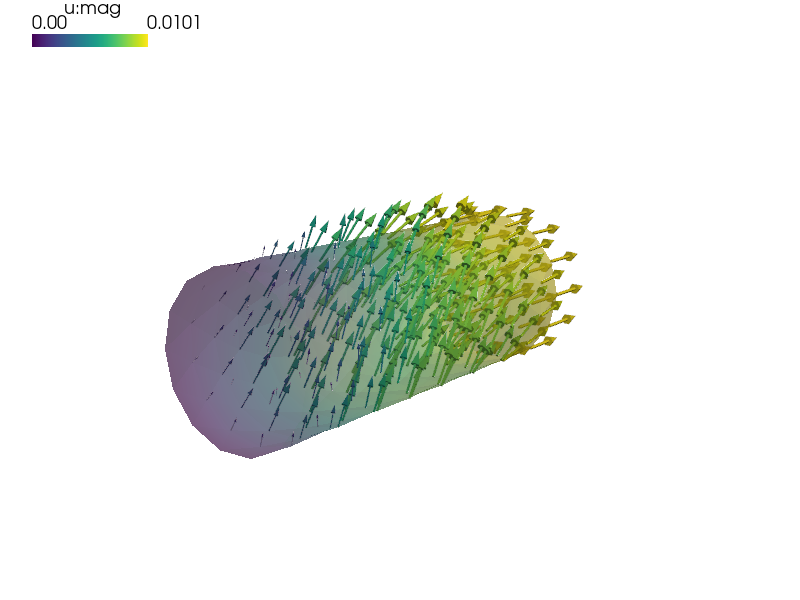# linear_elasticity/linear_elastic.py¶

Description

Linear elasticity with given displacements.

Findsuch that:whereThis example models a cylinder that is fixed at one end while the second end has a specified displacement of 0.01 in the x direction (this boundary condition is named 'Displaced'). There is also a specified displacement of 0.005 in the z direction for points in the region labeled 'SomewhereTop'. This boundary condition is named 'PerturbedSurface'. The region 'SomewhereTop' is specified as those vertices for which:

(z > 0.017) & (x > 0.03) & (x <  0.07)


The displacement field (three DOFs/node) in the 'Omega region' is approximated using P1 (four-node tetrahedral) finite elements. The material is linear elastic and its properties are specified as Lamé parametersand(see http://en.wikipedia.org/wiki/Lam%C3%A9_parameters)

The output is the displacement for each vertex, saved by default to cylinder.vtk. View the results using:

$./postproc.py cylinder.vtk --wireframe -b --only-names=u -d'u,plot_displacements,rel_scaling=1'source code # -*- coding: utf-8 -*- r""" Linear elasticity with given displacements. Find :math:\ul{u} such that: .. math:: \int_{\Omega} D_{ijkl}\ e_{ij}(\ul{v}) e_{kl}(\ul{u}) = 0 \;, \quad \forall \ul{v} \;, where .. math:: D_{ijkl} = \mu (\delta_{ik} \delta_{jl}+\delta_{il} \delta_{jk}) + \lambda \ \delta_{ij} \delta_{kl} \;. This example models a cylinder that is fixed at one end while the second end has a specified displacement of 0.01 in the x direction (this boundary condition is named 'Displaced'). There is also a specified displacement of 0.005 in the z direction for points in the region labeled 'SomewhereTop'. This boundary condition is named 'PerturbedSurface'. The region 'SomewhereTop' is specified as those vertices for which:: (z > 0.017) & (x > 0.03) & (x < 0.07) The displacement field (three DOFs/node) in the 'Omega region' is approximated using P1 (four-node tetrahedral) finite elements. The material is linear elastic and its properties are specified as Lamé parameters :math:\lambda and :math:\mu (see http://en.wikipedia.org/wiki/Lam%C3%A9_parameters) The output is the displacement for each vertex, saved by default to cylinder.vtk. View the results using::$ ./postproc.py cylinder.vtk --wireframe -b --only-names=u -d'u,plot_displacements,rel_scaling=1'
"""
from __future__ import absolute_import
from sfepy import data_dir
from sfepy.mechanics.matcoefs import stiffness_from_lame

filename_mesh = data_dir + '/meshes/3d/cylinder.mesh'

regions = {
'Omega' : 'all',
'Left' : ('vertices in (x < 0.001)', 'facet'),
'Right' : ('vertices in (x > 0.099)', 'facet'),
'SomewhereTop' : ('vertices in (z > 0.017) & (x > 0.03) & (x < 0.07)',
'vertex'),
}

materials = {
'solid' : ({'D': stiffness_from_lame(dim=3, lam=1e1, mu=1e0)},),
}

fields = {
'displacement': ('real', 'vector', 'Omega', 1),
}

integrals = {
'i' : 1,
}

variables = {
'u' : ('unknown field', 'displacement', 0),
'v' : ('test field', 'displacement', 'u'),
}

ebcs = {
'Fixed' : ('Left', {'u.all' : 0.0}),
'Displaced' : ('Right', {'u.0' : 0.01, 'u.[1,2]' : 0.0}),
'PerturbedSurface' : ('SomewhereTop', {'u.2' : 0.005}),
}

equations = {
'balance_of_forces' :
"""dw_lin_elastic.i.Omega(solid.D, v, u) = 0""",
}

solvers = {
'ls': ('ls.auto_direct', {}),
'newton': ('nls.newton', {
'i_max'      : 1,
'eps_a'      : 1e-10,
}),
}